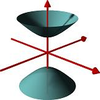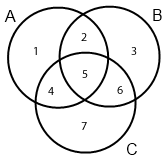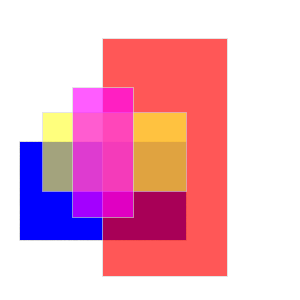#### You may also like### A Close Match

Can you massage the parameters of these curves to make them match as closely as possible?### Prime Counter

A short challenge concerning prime numbers.### The Right Volume

Can you rotate a curve to make a volume of 1?

# Venn Diagram Fun

##### Age 16 to 18 ShortChallenge Level

Consider the Venn diagram for 3 sets $A$, $B$ and $C$ with each of the $7$ regions labelled as followsThe set-theoretic representation of these regions is

1. $C^c\cap B^c$

2. $A\cap B \cap C^c$

3. $A^c \cap C^c$

4. $A\cap C \cap B^c$

5. $A\cap B\cap C$

6. $B\cap C \cap A^c$

7. $A^c \cap B^c$

A four-region diagram can be constructed by overlapping four rectangles (blue, pink, yellow, red) as follows:Note that there are $15$ distinct regions of the diagram, corresponding to $2^4-1$. Make sure your diagram has exactly 15 regions!